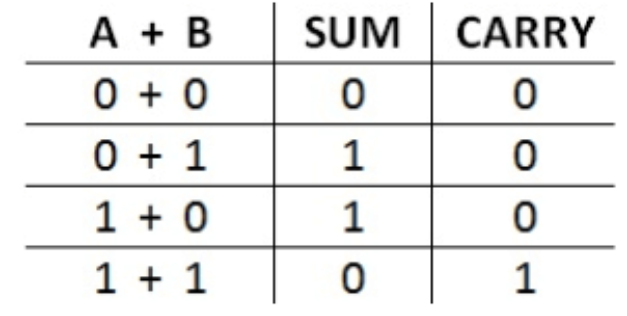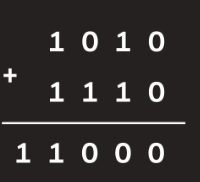### Program to add two Binary numbers

Description:
Binary numbers are the numbers which are expressed in the binary system or base 2 numeral system generally consisting of two numerical values 1 and 0.Binary addition is generally done as follows case -i If A=0 and B=0 then sum=0 and carry=0.
case-ii If A=0 and B=1 or A=1 and B=0 then the sum=1 and the carry=0.
case-iii If A=1 and B=1 then the sum=o and a carry=1 will be generated.Let us consider a example:From the above example we can assume the algorithm as
Algorithm: We need to check both the given numbers by traversing digits of both the numbers ,there would be 3 possible cases.
Case-1 if both the given numbers are zero that is A=0 and B=0.
if there is no previous carry then the answer would be 0
else if there is a previous carry then the answer would be 1.
Case-2 if one of the number is 1 and the other is 0 that is if A=0 and B=1 or A=1 and B=0.
if there is no previous carry then the answer would be 1.
else if there is a previous carry then the answer would be 0 and it would generate a further carry 1.
Case-3 if both the numbers are 1
if there is no previous carry then the answer would be 0.
else if there is a previous carry then the answer would be 0 and it would generate a further carry 1.
Code:
#include<iostream>
using namespace std;
int reverse(int n);/*function to reverse the number*/

int main(){
/*declaring two variables a and b to take two numbers as input*/
int a,b;
cout<<"Enter the value of the 1st Binary number";
cin>>a;
cout<<"Enter the value of the 2nd Binary number";
cin>>b;
/* printing the value returned by the function that is result or sum of the 2 binary numbers*/

}
/* variable to store the sum and initialising its value to zero*/
int ans=1;
/*variable to store the previous carry  and initialising its value to zero*/
int prevCarry=0;
while(a>0 && b>0){
/* coding the 3 cases learned above*/
if(a%2==0 && b%2==0){
ans=ans*10+prevCarry;
/*initialising prevCarry=0 because there is no prevCarry generated above*/
prevCarry=0;
}
else if((a%2==0 && b%2==1) || (a%2==1 && b%2==0)){
/*firstly we need to check if prevCarry was 0 or 1*/
if(prevCarry==1){
ans=ans*10+0;
prevCarry=1;
}
else{
ans=ans*10+1;
prevCarry=0;
}

}
else /* if both the numbers are 1*/
{
ans=ans*10+prevCarry;
prevCarry=1; /*since 1+1 always generates a carry 1*/
}
/* updating a and b values*/
a/=10;
b/=10;
}
/* if one of the bnumbers a or b has been finished traversing  that is the input numbers a and b are not of same lenght then we need to take care of the other number and add it with  the carry with the left out numbers*/
while(a>0){
if(prevCarry==1){
/* we need to check whether the number to which we are adding the prevCarry is 1 or not*/
if(a%2==1){
ans=ans*10 + 0;
prevCarry=1;/*since 1+1 generates a carry*/
}
else{
ans=ans*10 + 1;
prevCarry=0;/*initialising it to zero since the prevCarry has been utilized above*/

}
}
/* if prevCarry==0*/
else{
ans=ans*10 + (a%2);
}
/*updating value of a*/
a/=10;
}

/* same goes for b as well if b has been leftout */
while(b>0){
if(prevCarry==1){
/* we need to check whether the number to which we are adding the prevCarry is 1 or not*/
if(b%2==1){
ans=ans*10 + 0;
prevCarry=1;/*since 1+1 generates a carry*/
}
else{
ans=ans*10 + 1;
prevCarry=0;/*initialising it to zero since the prevCarry has been utilized above*/

}
}
/* if prevCarry==0*/
else{
ans=ans*10 + (b%2);
}
/*updating value of b*/
b/=10;
}
/* if and b values are of same length that is input numbers are equal then atlast if we are leftout with a carry*/
if(prevCarry==1){
ans= ans*10+1;
}
/* we need to reverse the result because we are adding the numbers here since we are adding numbers by using the %10 logic the first number will become the last number so we need to reverse  the number*/
/*function to reverse the number*/
ans =reverse(ans);
return ans;
}

int reverse(int n){/*declaring n as a parameter to store the value of the answer from the above function*/
int ans=0;/* declaring  variable to store the reverse number and initialising it to 0 */
while(n>0){
int lastnum=n%10;
ans=ans*10+lastnum;
n/=10;/*updating n value*/
}
/*returning value to the above function where reverse function has been called*/
ans=ans/10 ;
return ans;
}#### More Articles of Shaik Aftab Ahmed:

Name Views Likes
C++ Program to Find the Frequency of Odd & Even Numbers in the given Matrix 394 1
C++ program to Sort a Linked List Using Merge Sort 358 1
C++ Program to Implement a Linked List representation of a Binary tree 353 1
C++ Program to Check for balanced parentheses in an expression 250 1
C++ Program to Perform Inorder, Preorder, Postorder traversals in a binary tree. 289 1
C++ program to print Indian flag 385 1
C++ program to Convert a multi level linked list to a singly linked list 269 1
C++ program to print right view of a Binary tree using queue 243 1
C++ Program to implement Huffman Coding Compression Algorithm 1657 1
C++ Program to Create a Height Balanced Binary Tree 274 1
C++ program to implement Prims algorithm 635 1
C++ Program for BFS Traversal 288 1
C++ Progam to Evaluate a Prefix Expression 460 1
C++ Program to Implement Queue using Linked List 254 1
C++ implementation of Stack using Linked list 303 1
C++ program to find the intersection point of two linked lists 338 1
C++ program to count the inversions in the given array 278 1
C++ program to perform D.N.F sort 325 1
C++ program to print all possible subsets of a String 286 1
C++ program to count the number of ones in a binary representation of a number 310 1
C++ program to print all possible subsets of a set 322 1
C++ program to find the largest word in a String 287 1
C++ Program to print a matrix in Spiral order 365 1
C++ program to convert from Binary to Decimal, Octal to Decimal, Hexadecimal to Decimal 312 1
C limits library 340 1
Program to add two Binary numbers 332 1